# Maharashtra Board Miscellaneous Problems Set 1 Class 7 Maths Solutions

Balbharti Maharashtra State Board Class 7 Maths Solutions covers the 7th Std Maths Miscellaneous Problems Set 1 Answers Solutions.

## Maharashtra Board Miscellaneous Problems Set 1 Class 7 Maths Solutions

Question 1.
Solve the following:
i. (-16) × (-5)
ii. (72) ÷ (-12)
iii. (-24) × (2)
iv. 125 ÷ 5
v. (-104) ÷ (-13)
vi. 25 × (-4)
Solution:
i. (-16) × (-5) = 80

ii. 72 ÷ (-12) = $$\frac { 72 }{ -12 }$$
= $$\frac{1}{(-1)} \times \frac{72}{12}$$
(-1) × 12
= -6

iii. (-24) × 2 = -48

iv. 125 ÷ 5 = $$\frac { 125 }{ 5 }$$
= 25

v. (-104) ÷ (-13) = $$\frac { -104 }{ -13 }$$
= $$\frac { 104 }{ 13 }$$
= 8

vi. 25 × (-4) = -100

Question 2.
Find the prime factors of the following numbers and find their LCM and HCF:
i. 75,135
ii. 114,76
iii. 153,187
iv. 32,24,48
Solution:
i. 75 = 3 × 25
= 3 × 5 × 5
135 = 3 × 45
= 3 × 3 × 15
= 3 × 3 × 3 × 5
∴ HCF of 75 and 135 = 3 × 5
= 15
LCM of 75 and 135 = 3 × 5 × 5 × 3 × 3
= 675

ii. 114 = 2 × 57
= 2 × 3 × 19
76 = 2 × 38
= 2 × 2 × 19
∴ HCF of 114 and 76 = 2 × 19
= 38
LCM of 114 and 76 = 2 × 19 × 3 × 2
= 228

iii. 153 = 3 × 51
= 3 × 3 × 17
187 = 11 × 17
∴ HCF of 153 and 187 = 17
LCM of 153 and 187 = 17 × 3 × 3 × 11
= 1683

iv. 32 = 2 × 16
= 2 × 2 × 8
= 2 × 2 × 2 × 4
= 2 × 2 × 2 × 2 × 2
24 = 2 × 12
= 2 × 2 × 6
= 2 × 2 × 2 × 3
48 = 2 × 24
= 2 × 2 × 12
= 2 × 2 × 2 × 6
= 2 × 2 × 2 × 2 × 3
∴ HCF of 32, 24 and 48 = 2 × 2 × 2
= 8
LCM of 32,24 and 48 = 2 × 2 × 2 × 2 × 2 × 3
= 96

Question 3.
Simplify:
i. $$\frac { 322 }{ 391 }$$
ii. $$\frac { 247 }{ 209 }$$
iii. $$\frac { 117 }{ 156 }$$
Solution:
i. $$\frac { 322 }{ 391 }$$ii. $$\frac { 247 }{ 209 }$$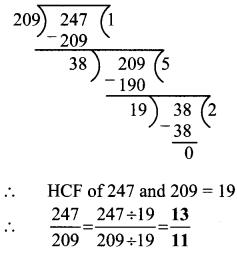iii. $$\frac { 117 }{ 156 }$$Question 4.
i. 784
ii. 225
iii. 1296
iv. 2025
v. 256
Solution:
i. 784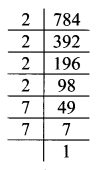∴ 784 = 2 × 2 × 2 × 2 × 7 × 7
∴ √784 = 2 × 2 × 7
= 28

ii. 225∴ 225 = 3 × 3 × 5 × 5
∴ √225 = 3 × 5
= 15

iii. 1296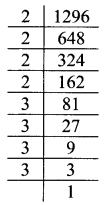∴ 1296 = 2 × 2 × 2 × 2 × 3 × 3 × 3 × 3
∴ √1296 = 2 × 2 × 3 × 3
= 36

iv. 2025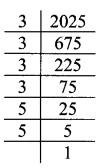∴ 2025 = 3 × 3 × 3 × 3 × 5 × 5
∴ √2025 = 3 × 3 × 5
= 45

v. 256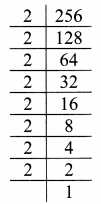∴ 256 = 2 × 2 × 2 × 2 × 2 × 2 × 2 × 2
∴ √256 = 2 × 2 × 2 × 2
= 16

Question 5.
There are four polling booths for a certain election. The numbers of men and women who cast their vote at each booth is given in the table below. Draw a joint bar graph for this data.

 Polling Booths Navodaya Vidyalaya Vidyaniketan School City High School Eklavya School Women 500 520 680 800 Men 440 640 760 600

Solution: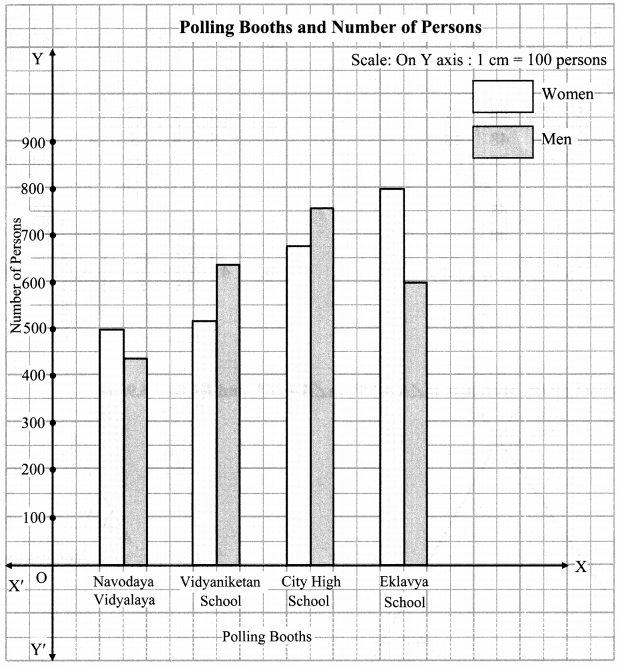Question 6.
Simplify the expressions:
i. 45 ÷ 5 + 120 × 4 – 12
ii. (38 – 8) × 2 ÷ 5 + 13
iii. $$\frac{5}{3}+\frac{4}{7} \div \frac{32}{21}$$
iv. 3 × {4 [85 + 5 – (15 – 3)] + 2}
Solution:
i. 45 ÷ 5 + 120 × 4 – 12
= 9 + 80 – 12
= 89 – 12
= 77

ii. (38 – 8) × 2 ÷ 5 + 13
= 30 × 2 ÷ 5 + 13
= 60 ÷ 5 + 13
= 12 + 13
= 25

iii. $$\frac{5}{3}+\frac{4}{7} \div \frac{32}{21}$$
$$\frac{5}{3}+\frac{4}{7} \times \frac{21}{32}$$
$$\frac{5}{3}+\frac{3}{8}=\frac{40}{24}+\frac{9}{24}$$
$$\frac{49}{24}$$

iv. 3 × {4 [85 + 5 – (15 – 3)] + 2}
= 3 × {4[90 – 5] + 2}
= 3 × {4 × 85 + 2}
= 3 × (340 + 2)
= 3 × 342
= 1026

Question 7.
Solve:
i. $$\frac{5}{12}+\frac{7}{16}$$
ii. $$3 \frac{2}{5}-2 \frac{1}{4}$$
iii. $$\frac{12}{5} \times \frac{(-10)}{3}$$
iv. $$4 \frac{3}{8} \div \frac{25}{18}$$
Solution:
i. $$\frac{5}{12}+\frac{7}{16}$$ii. $$3 \frac{2}{5}-2 \frac{1}{4}$$iii. $$\frac{12}{5} \times \frac{(-10)}{3}$$
= 4 × (-2)
= -8

iv. $$4 \frac{3}{8} \div \frac{25}{18}$$
= $$\frac{7}{4} \times \frac{9}{5}$$
= $$\frac { 63 }{ 20 }$$

Question 8.
Construct ∆ABC such that m∠A = 55°, m∠B = and l(AB) = 5.9 cm.
Solution: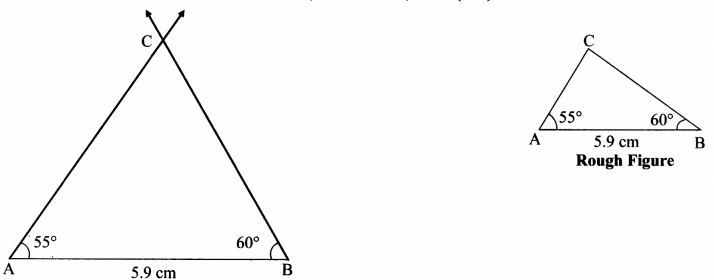Question 9.
Construct ∆XYZ such that, l(XY) = 3.7 cm, l(YZ) = 7.7 cm, l(XZ) = 6.3 cm.
Solution:Question 10.
Construct ∆PQR such that, m∠P = 80°, m∠Q = 70°, l(QR) = 5.7 cm.
Ans:
In ∆PQR,
m∠P + m∠Q + m∠R = 180° …. (Sum of the measures of the angles of a triangle is 180°)
∴ 80 + 70 + m∠R = 180
∴ 150 + m∠R = 180
∴ m∠R = 180 – 150
∴ m∠R = 30°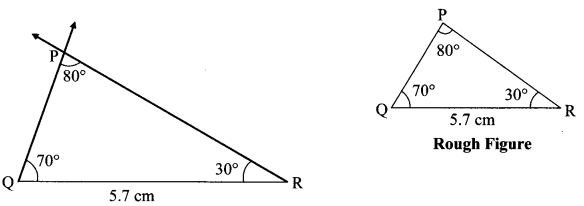Question 11.
Construct ∆EFG from the given measures. l(FG) = 5 cm, m∠EFG = 90°, l(EG) = 7 cm.
Solution:Question 12.
In ∆LMN, l(LM) = 6.2 cm, m∠LMN = 60°, l(MN) 4 cm. Construct ∆LMN.
Solution:Question 13.
Find the measures of the complementary angles of the following angles:
i. 35°
ii. a°
iii. 22°
iv. (40 – x)°
Solution:
i. Let the measure of the complementary
angle be x°.
35 + x = 90
∴35 + x-35 = 90 – 35
….(Subtracting 35 from both sides)
∴x = 55
∴The complementary angle of 35° is 55°.

ii. Let the measure of the complementary angle be x°.
a + x = 90
∴a + x – a = 90 – a
….(Subtracting a from both sides)
∴x = (90 – a)
∴The complementary angle of a° is (90 – a)°.

iii. Let the measure of the complementary angle be x°.
22 + x = 90
∴22 + x – 22 = 90 – 22
….(Subtracting 22 from both sides)
∴x = 68
∴The complementary angle of 22° is 68°.

iv. Let the measure of the complementary angle be a°.
40 – x + a = 90
∴40 – x + a – 40 + x = 90 – 40 + x
….(Subtracting 40 and adding x on both sides)
∴a = (50 + x)
∴The complementary angle of (40 – x)° is (50 + x)°.

Question 14.
Find the measures of the supplements of the following angles:
i. 111°
ii. 47°
iii. 180°
iv. (90 – x)°
Solution:
i. Let the measure of the supplementary
angle be x°.
111 + x = 180
∴ 111 + x – 111 = 180 – 111
…..(Subtracting 111 from both sides)
∴ x = 69
∴ The supplementary angle of 111° is 69°.

ii. Let the measure of the supplementary angle be x°.
47 + x = 180
∴47 + x – 47 = 180 – 47
….(Subtracting 47 from both sides)
∴x = 133
∴The supplementary angle of 47° is 133°.

iii. Let the measure of the supplementary angle be x°.
180 + x = 180
∴180 + x – 180 = 180 – 180
….(Subtracting 180 from both sides)
∴x = 0
∴The supplementary angle of 180° is 0°.

iv. Let the measure of the supplementary angle be a°.
90 – x + a = 180
∴90 – x + a – 90 + x = 180 – 90+ x
….(Subtracting 90 and adding x on both sides)
∴a = 180 – 90 + x
∴a = (90 + x)
∴The supplementary angle of (90 – x)° is (90 + x)°.

Question 15.
Construct the following figures:
i. A pair of adjacent angles
ii. Two supplementary angles which are not adjacent angles.
iii. A pair of adjacent complementary angles.
Solution:
i.ii.iii.Question 16.
In ∆PQR the measures of ∠P and ∠Q are equal and m∠PRQ = 70°, Find the measures of the following angles.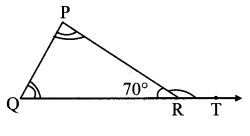1. m∠PRT
2. m∠P
3. m∠Q

Solution:
Here, ∠PRQ and ∠PRT are angles in a linear pair.
m∠PRQ + m∠PRT = 180°
∴70 + m∠PRT = 180
∴m∠PRT = 180 – 70
∴m∠PRT = 110°
Now, ∠PRT is the exterior angle of ∆PQR.
∴m∠P + m∠Q = m∠PRT
∴m∠P + m∠P = m∠PRT ….(The measures of ∠P and ∠Q is same)
∴2m∠P = 110
∴m∠P = $$\frac { 110 }{ 2 }$$
∴m∠P = 55°
∴m∠Q =

Question 17.
Simplify
i. 54 × 53
ii. $$\left(\frac{2}{3}\right)^{6} \div\left(\frac{2}{3}\right)^{9}$$
iii. $$\left(\frac{7}{2}\right)^{8} \times\left(\frac{7}{2}\right)^{-6}$$
iv. $$\left(\frac{4}{5}\right)^{2} \div\left(\frac{5}{4}\right)$$
Solution:
Simplify
i. 54 × 53
= 54+3
= 57

ii. $$\left(\frac{2}{3}\right)^{6} \div\left(\frac{2}{3}\right)^{9}$$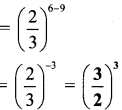iii. $$\left(\frac{7}{2}\right)^{8} \times\left(\frac{7}{2}\right)^{-6}$$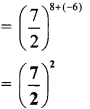iv. $$\left(\frac{4}{5}\right)^{2} \div\left(\frac{5}{4}\right)$$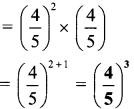Question 18.
Find the value:
i. 1716  ÷ 1716
ii. 10-3
iii. (2³)²
iv. 46 × 4-4
Solution:
i. 1716  ÷ 1716
= 170
= 1

ii. 10-3
= $$\frac{1}{10^{3}}$$
= $$\frac{1}{1000}$$

iii. (2³)²
= 23×2
= 26
= 2 × 2 × 2 × 2 × 2 × 2
= 64

iv. 46 × 4-4
= 46+(-4)
= 42
= 4 × 4
= 16

Question 19.
Solve:
i. (6a – 5b – 8c) + (15b + 2a – 5c)
ii. (3x + 2y) (7x – 8y)
iii. (7m – 5n) – (-4n – 11m)
iv. (11m – 12n + 3p) – (9m + 7n – 8p)
Solution:
i. (6a – 5b – 8c) + (15b + 2a – 5c)
= (6a + 2a) + (-5b + 15b) + (-8c – 5c)
= 8a + 10b – 13c

ii. (3x + 2y) (7x – 8y)
= 3x × (7x – 8y) + 2yx (7x – 8y)
= 21x² – 24xy + 14xy – 16y²
= 21x² – 10xy – 16y²

iii. (7m – 5n) – (-4n – 11m)
= 7m – 5n + 4n + 11m
= (7m + 11m) + (-5n + 4n)
= 18m – n

iv. (11m – 12n + 3p) – (9m + 7n – 8p)
= 11m – 12n + 3p – 9m – 7n + 8p
= (11m – 9m) + (-12n – 7n) + (3p + 8p)
= 2m – 19n + 11p

Question 20.
Solve the following equations:
i 4(x + 12) = 8
ii. 3y + 4 = 5y – 6
Solution:
i. 4(x + 12) = 8
∴4x + 48 = 8
∴4x + 48 – 48 = 8 – 48
….(Subtracting 48 from both sides)
∴ 4x = -40
∴ x = $$\frac { -40 }{ 4 }$$
∴ x = -10

ii. 3y + 4 = 5y – 6
∴ 3y + 4 + 6 = 5y – 6 + 6
∴ y = $$\frac { 10 }{ 2 }$$